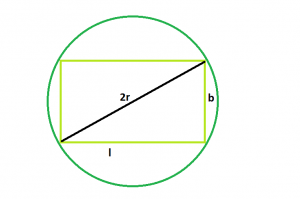Here we have a rectangle of length l & breadth b.We have to find the circumradius of the rectangle.

Examples:

```Input : l = 3, b = 4
Output :2.5

Input :l = 10, b = 12
Output :3.95227774224
```

## Recommended: Please try your approach on {IDE} first, before moving on to the solution.Approach:
From the diagram, we can clearly understand the circumradius r is half of the diagonal of the rectangle.

r = √(l^2 + b^2)/2

Below is the implementation of the above approach:

## C++

 `// C++ Program to find the radius ` `// of the circumcircle of the given rectangle ` ` `  `#include ` `using` `namespace` `std; ` ` `  `// Function to find the radius ` `// of the circumcircle ` `float` `findRadiusOfcircumcircle(``float` `l, ``float` `b) ` `{ ` ` `  `    ``// the sides cannot be negative ` `    ``if` `(l < 0 || b < 0) ` `        ``return` `-1; ` ` `  `    ``// Radius of the circumcircle ` `    ``float` `radius = ``sqrt``(``pow``(l, 2) + ``pow``(b, 2)) / 2; ` ` `  `    ``// Return the radius ` `    ``return` `radius; ` `} ` ` `  `// Driver code ` `int` `main() ` `{ ` ` `  `    ``// Get the sides of the traingle ` `    ``float` `l = 4, b = 3; ` `    ``// Find the radius of the circumcircle ` `    ``cout << findRadiusOfcircumcircle(l, b) << endl; ` ` `  `    ``return` `0; ` `} `

## Java

 `// Java Program to find the radius ` `// of the circumcircle of the given ` `// rectangle ` `import` `java.util.*; ` `import` `java.lang.*; ` `import` `java.io.*; ` ` `  `class` `GFG ` `{ ` ` `  `// Function to find the radius ` `// of the circumcircle ` `static` `float` `findRadiusOfcircumcircle(``float` `l, ` `                                      ``float` `b) ` `{ ` ` `  `    ``// the sides cannot be negative ` `    ``if` `(l < ``0` `|| b < ``0``) ` `        ``return` `-``1``; ` ` `  `    ``// Radius of the circumcircle ` `    ``float` `radius = (``float``) Math.sqrt(Math.pow(l, ``2``) + ` `                           ``Math.pow(b, ``2``)) / ``2``; ` ` `  `    ``// Return the radius ` `    ``return` `radius; ` `} ` ` `  `// Driver code ` `public` `static` `void` `main(String args[]) ` `{ ` ` `  `    ``// Get the sides of the traingle ` `    ``float` `l = ``4``, b = ``3``; ` `    ``// Find the radius of the circumcircle ` `    ``System.out.println(findRadiusOfcircumcircle(l, b)); ` `} ` `} ` ` `  `// This code is contributed by Subhadeep `

## Python3

 `# Python Program to find the  ` `# radius of the circumcircle ` `# of the given rectangle ` `import` `math  ` ` `  `# Function to find the radius ` `# of the circumcircle ` `def` `findRadiusOfcircumcircle(l, b): ` ` `  `    ``# the sides cannot be negative ` `    ``if` `(l < ``0` `or` `b < ``0``): ` `        ``return` `-``1``; ` ` `  `    ``# Radius of the circumcircle ` `    ``radius ``=` `(math.sqrt(``pow``(l, ``2``) ``+`  `                        ``pow``(b, ``2``)) ``/` `2``); ` ` `  `    ``# Return the radius ` `    ``return` `radius; ` ` `  `# Driver code ` ` `  `# Get the sides of the traingle ` `l ``=` `4``; ` `b ``=` `3``; ` `     `  `# Find the radius of the circumcircle ` `print``(findRadiusOfcircumcircle(l, b)); ` ` `  `# This code is contributed  ` `# by Shivi_Aggarwal `

## C#

 `// C# Program to find the radius ` `// of the circumcircle of the  ` `// given rectangle ` `using` `System; ` ` `  `class` `GFG ` `{ ` ` `  `// Function to find the radius ` `// of the circumcircle ` `static` `float` `findRadiusOfcircumcircle(``float` `l, ` `                                       ``float` `b) ` `{ ` ` `  `    ``// the sides cannot be negative ` `    ``if` `(l < 0 || b < 0) ` `        ``return` `-1; ` ` `  `    ``// Radius of the circumcircle ` `    ``float` `radius = (``float``) Math.Sqrt(Math.Pow(l, 2) + ` `                           ``Math.Pow(b, 2)) / 2; ` ` `  `    ``// Return the radius ` `    ``return` `radius; ` `} ` ` `  `// Driver code ` `public` `static` `void` `Main() ` `{ ` ` `  `    ``// Get the sides of the traingle ` `    ``float` `l = 4, b = 3; ` `     `  `    ``// Find the radius of the circumcircle ` `    ``Console.WriteLine(findRadiusOfcircumcircle(l, b)); ` `} ` `} ` ` `  `// This code is contributed by anuj_67 `

## PHP

 ` `

Output:

`2.5`

Attention reader! Don’t stop learning now. Get hold of all the important DSA concepts with the DSA Self Paced Course at a student-friendly price and become industry ready.

My Personal Notes arrow_drop_upBudding Web DeveloperKeen learnerAverage CoderDancer&Social Activist

If you like GeeksforGeeks and would like to contribute, you can also write an article using contribute.geeksforgeeks.org or mail your article to contribute@geeksforgeeks.org. See your article appearing on the GeeksforGeeks main page and help other Geeks.

Please Improve this article if you find anything incorrect by clicking on the "Improve Article" button below.

Article Tags :
Practice Tags :

Be the First to upvote.

Please write to us at contribute@geeksforgeeks.org to report any issue with the above content.